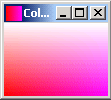Tutorials

A color gradient consisting of two or more colors blending together. To display the icon, we have defined the height and width of an image.

A color gradient consisting of two or more colors blending together. To display the icon, we have defined the height and width of an image.

This section illustrates you how the icon shows the Color Gradient.

A color gradient consisting of two or more colors blending together. To display the icon, we have defined the height and width of an image. To produce pixel values for an Image, we have used the class MemoryImageSource which is an implementation of the interface ImageProducer

The method getPreferredSize() is used to determine the arrangement of layout and indicates the preferred size.

Following code draws the image:

 g.drawImage(image, 0, 0, getSize().width, getSize().height, this)

Here is the code of IconShowingColorGradient.java

 ``` import java.awt.*; import java.awt.image.MemoryImageSource; public class IconShowingColorGradient extends Component {   public static void main(String[] args) {   Frame frame = new Frame("Color Gradient");   frame.add(new IconShowingColorGradient());   frame.setIconImage(new IconShowingColorGradient(16, 16).getImage());   frame.setSize(100, 100);   frame.show();   } private Image image; private int width; private int height; public IconShowingColorGradient() {   this(100, 100);   } public IconShowingColorGradient(int w, int h) {   this.width = w;   this.height = h;   int pixel[] = new int[w * h];   int ind = 0;   for (int x = 0; x < h; x++) {   int color1 = (x * 255) / (h - 1);   for (int y = 0; y < w; y++) {   int color2 = (y * 255) / (w - 1);   pixel[ind++] = (255<<16) | (color1 << 24) | color2;   }   }   image = createImage(new MemoryImageSource(w, h, pixel, 0,w));   setSize(getPreferredSize());   } public Image getImage() {   return image;   } public Dimension getPreferredSize() {   return new Dimension(width, height);   } public void paint(Graphics g) {   g.drawImage(image, 0, 0, getSize().width, getSize().height, this);   } }```

Output will be displayed as